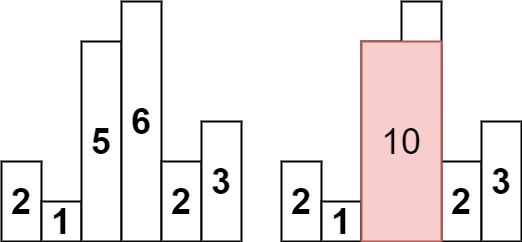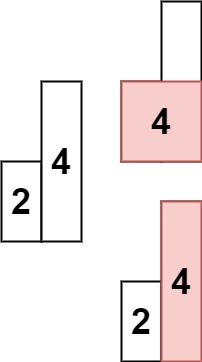# 84. Largest Rectangle in Histogram

Given an array of integers `heights` representing the histogram's bar height where the width of each bar is `1`, return the area of the largest rectangle in the histogram.

Example 1:`Input: heights = [2,1,5,6,2,3]`
`Output: 10`
```Explanation: The above is a histogram where width of each bar is 1.
The largest rectangle is shown in the red area, which has an area = 10 units.
```

Example 2:`Input: heights = [2,4]`
```Output: 4
```

Constraints:

• `1 <= heights.length <= 105`
• `0 <= heights[i] <= 104`

`Solution:`

`Prerquisite: Next Greater Element`

The first thing which comes to our mind is that we can traverse the given array and at every position, we can find the left smaller element and right smaller element. The area between these two will be the maximum area of the rectangle of height arr[i].

Now how we can use stack to know these things? Let's see.

We need to have the left smaller and the right smaller for every value at a particular index. For the left smaller we can start from the index 0 and we will also keep a stack. If the value at the top of the stack is greater than the present value we will simply pop it, and we will do this until we find a smaller number or the stack becomes empty.

while(!st.empty() && v[st.top()]>=v[i])st.pop();

If the stack is empty then we know that there is no left smaller and the boundary can be 0.

Similarly for the right smaller or next smaller we will start from the last index and will keep the same condition.

Now, this approach has extra spaces, and also we had two passes to get the left and the right smaller and then we calculated the answer. So how we can optimize this also? Let's think.

We will maintain a monotonic stack here and we will get the left and the right smaller from here only. If the current element is lesser than the top of the element in the stack then we found the right smaller for the top of the stack. And we also know that the stack is monotonic so we can get the left smaller by popping the top element. In this way, we got the left and right smaller from a single stack and single pass. But what if the stack becomes empty, if the stack becomes empty then we don't have the left smaller. Also at last we need to check if the stack has some elements, if yes then we need to calculate for them also. So for the left-out elements in the stack, we don't have the right smaller.

Now let's see the code for this approach. This can be tricky to understand.